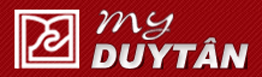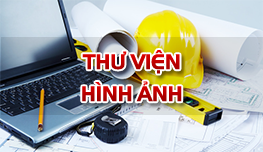Tài liệu học tập

### Bài tập SBVL1 (Tiếng Anh) - Phần TENSION, COMPRESSION and SHEAR (Bài 1 dến bài 5)

• Đăng bởi: lethithanhbinh
• Sinh viên
• 18/10/2018

Tension, compression and shear 1:

A short post constructed from a hollow circular tube of aluminum supports a compressive load of 26 kips (Fig. 1). The inner and outer diameters of the tube are d1 = 4.0 in. and d2 =  4.5 in., respectively, and its length is 16 in. The shortening of the post due to the load is measured as 0.012 in.  Determine the compressive stress and strain in the post.

Tension, compression and shear  2:

A circular steel rod of length L and diameter d hangs in a mine shaft and holds an ore bucket of weight W at its lower end (Fig. 2).

(a) Obtain a formula for the maximum stress smax in the rod, taking into account the weight of the rod itself.

(b) Calculate the maximum stress if L = 40 m, d = 8 mm, and W = 1.5 kN.

Tension, compression and shear 3:

A steel pipe of length L = 4.0 ft, outside diameter d2 =  6.0 in., and inside diameter d1 =  4.5 in. is compressed by an axial force P = 140 k (Fig. 3). The material has modulus of elasticity E = 30,000 ksi and Poisson’s ratio n = 0.30.  Determine the following quantities for the pipe: (a) the shortening d, (b) the lateral strain e’, (c) the increase Dd2 in the outer diameter and the increase Dd1 in the inner diameter, and (d) the increase Dt in the wall thickness.

Tension, compression and shear 4:

A punch for making holes in steel plates is shown in Fig. 4a. Assume that a punch having diameter d =  20 mm is used to punch a hole in an 8-mm plate, as shown in the cross-sectional view (Fig. 4b). If a force P = 110 kN is required to create the hole, what is the average shear stress in the plate and the average compressive stress in the punch?

Tension, compression and shear 5

A steel strut S serving as a brace for a boat hoist transmits a compressive force P = 12 k to the deck of a pier (Fig. 5a). The strut has a hollow square cross section with wall thickness t = 0.375 in. (Fig. 5b), and the angle u between the strut and the horizontal is 40° . A pin through the strut transmits the compressive force from the strut to two gussets G that are welded to the base plate B. Four anchor bolts fasten the base plate to the deck.  The diameter of the pin is dpin  = 0.75 in., the thickness of the gussets is tG = 0.625 in., the thickness of the base plate is tB = 0.375 in., and the diameter of the anchor bolts is dbolt  = 0.50 in. Determine the following stresses: (a) the bearing stress between the strut and the pin, (b) the shear stress in the pin, (c) the bearing stress between the pin and the gussets, (d) the bearing stress between the anchor bolts and the base plate, and (e) the shear stress in the anchor bolts. (Disregard any friction between the base plate and the deck.)

Tải file đính kèm:

Bài viết liên quan